The Transistor Amplifier TEST Home Save as: .doc (1.4MB)     .pdf (1MB) See: 1- 100 Transistor Circuits 101 - 200 Transistor Circuits P1   P2   P3

 Read P1 and  P2 of The Transistor Amplifier before answering these TEST questions:

 Question 1: What is the name of this stage: (circuit)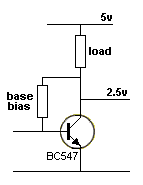Mouseover for answer

 Question 2: What is the name of this stage: (circuit)Mouseover for answer

 Question 3: Identify the common-base stage: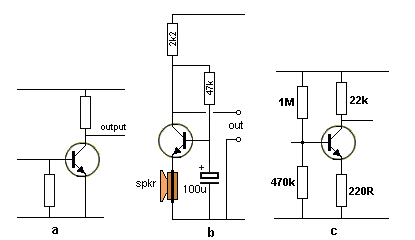Mouseover for answer

 Question 4: What is the full name give to this type of stage: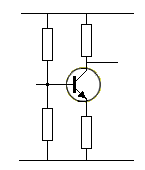Mouseover for answer

 Question 5: What is the name of this stage:Mouseover for answer

 Question 6: When the signal is rising on the input of this stage, what happens to the output signal:Mouseover for answer

 Question 7: To achieve the highest amplification with the least distortion, what is the approximate voltage on the collector, in quiescent mode (rest mode):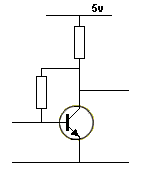Mouseover for answer

 Question 8: Describe this stage fully:Mouseover for answer

 Question 9:What is the approximate voltage-gain you will get from this stage:Mouseover for answer

 Question 10: What is the approximate voltage-gain you will get from this stage: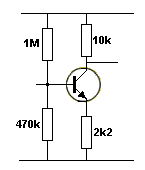Mouseover for answer

 Question 11: Describe this circuit:Mouseover for answer

 Question 12: Describe this circuit:Mouseover for answer

 Question 13: Identify the feedback component . Does the component provide Positive Feedback or Negative Feedback?Mouseover for answer

 Question 14: Identify the feedback component. Does the component provide Positive Feedback or Negative Feedback?Mouseover for answer

 Question 15: What is the name of this circuit: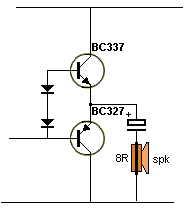Mouseover for answer

 Question 16: If the motor takes 300mA, what is the input current:   10mA  or  1mA   or  0.1mA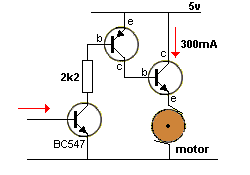Mouseover for answer

 Question 17: Name this circuit: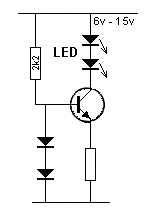Mouseover for answer

 Question 18: Name this circuit: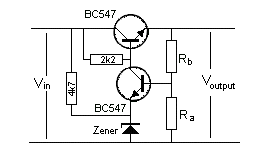Mouseover for answer

 Question 19: Identify the feedback component in this TOUCH SWITCH circuit. Does it provide Positive Feedback or Negative Feedback?Mouseover for answer

 Question 20: Which switch will turn on the 12v globe: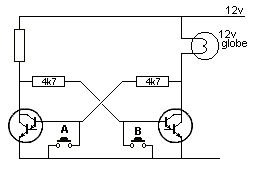Mouseover for answer

 Question 21: Identify this circuit: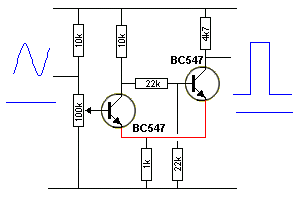Mouseover for answer

 Question 22: Identify this circuit:Mouseover for answer

 Question 23: Identify these two circuits: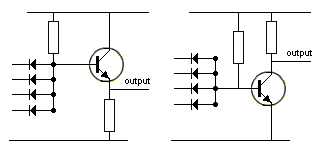Mouseover for answer

 Question 24: Is the transistor SINKING or SOURCING the current: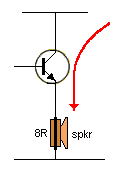Mouseover for answer

 Question 25: Name this circuit: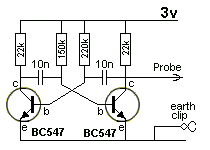Mouseover for answer

 Question 26: Name this circuit:Mouseover for answer

 Question 27: Describe the output voltage when the input voltage changes from 0v to  -5volts:Mouseover for answer

 Question 27a: Describe the output voltage when the input voltage changes from 0v to  10volts:Mouseover for answer

 Question 28: What is the voltage on the base of stages (A)  (B) and (C):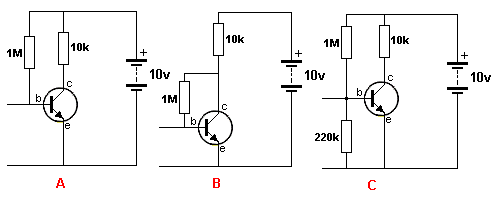Mouseover for answer

 Question 29: Describe the output voltage as the voltage from the pot is increased: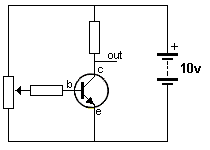Mouseover for answer

 Question 30: If Rb is removed, the transistor is (a) saturated,  (b) non-conducting (c) cut-off:Mouseover for answer

 Question 31: Name the negative feedback component: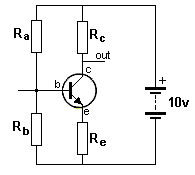Mouseover for answer

 Question 32: What is the purpose of the 47R resistor: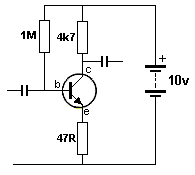Mouseover for answer

 Question 33: Name this type of circuit: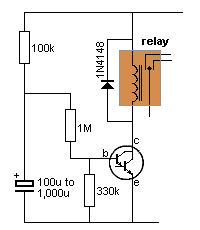Mouseover for answer

 Question 34: What is the purpose of the zener diode:Mouseover for answer

 Question 35: Name this type of circuit:Mouseover for answer

 Question 36: If the transistor zeners at 45v, what is the maximum voltage across the 1u electrolytic in Q35: Mouseover for answer

 Question 37: Name this circuit:Mouseover for answer

 Question 38: Does this circuit produce a clean audio output or an "over-driven" digital output: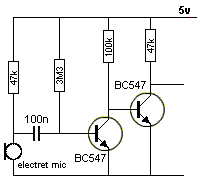Mouseover for answer

 Question 39: When the transistor receives an  AC signal, does the 10u charge or discharge: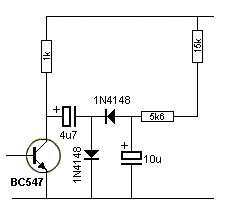Mouseover for answer

 Question 40: Does the transistor pull in two directions:Mouseover for answer

 Question 41: Does the 1N4004 diode remove any spikes from the supply?Mouseover for answer

 Question 42: When the transistor turns off, the voltage on the lower end of the inductor will be: (a) 9v   (b) 7v  (c) 0v   (d) greater than 9v.Mouseover for answer